# Schedule of Classes September 3 September 10 September

• Slides: 23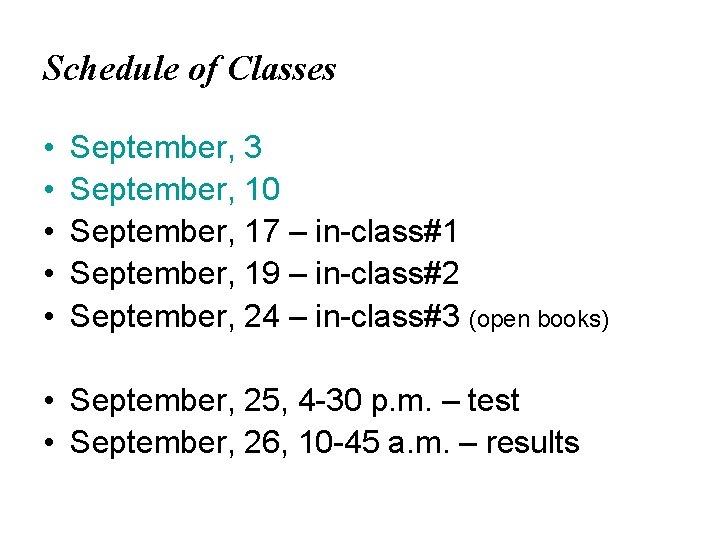Schedule of Classes • • • September, 3 September, 10 September, 17 – in-class#1 September, 19 – in-class#2 September, 24 – in-class#3 (open books) • September, 25, 4 -30 p. m. – test • September, 26, 10 -45 a. m. – resultsTopic 2. Demand Supply Topic 2. 1. Individual Consumer DemandUtility • The decision to buy is based upon two considerations: – The utility that you derive from the commodity – The ability to pay for it • Def. : Utility – the pleasure or satisfaction associated with having, using, or consuming goods or services – The cardinal approach to consumer equilibrium – The ordinal approach to consumer equilibrium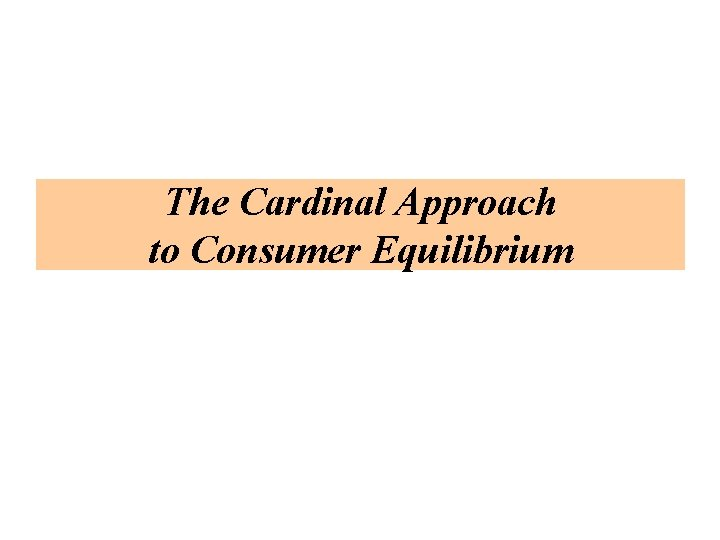The Cardinal Approach to Consumer EquilibriumUtility Function • Utility can be measured in units called utils • A utility function is obtained by attaching a number to each market basket, so that if market basket is preferred to market basket B, the number will be higher for A than for BMarginal Utility • Marginal utility is defined as the change in total utility that results from a one-unit change in consumptionThe Law of Diminishing Marginal Utility • Marginal utility diminishes as quantity of the commodity consumed increases • The assumption of diminishing marginal utility is one of the most important cornerstones of economic theory • The saturation point at which TU is maximum is determined as the point where MU=0The Model of Consumer Behavior (Assumptions) • Consumers are free to spend their incomes as they please • Consumers have perfect knowledge of all factors that may affect their decision • The sales units of commodities are divisible • The consumer’s tastes and preferences are well established • The marginal utility of each commodity diminishes for the consumer as the quantity consumed increases • More is better than less • Consumers always attempt to maximize utilityConsumer Equilibrium at Maximum Utility • ? : How does a consumer decide what to buy? – Consumers always try to get the most for their money • Maximum utility is a position of equilibrium • The cost of consumption (the utility of money) is balanced against the utility to be gained from the purchase (see next slide)Consumer’s Equilibrium • Each commodity purchased provides marginal utility that diminishes as consumption increases – and each can be purchased at a particular price • At this point the consumer can no longer increase total utility by buying more or less – The marginal utility per last dollar spent is equal for all commodities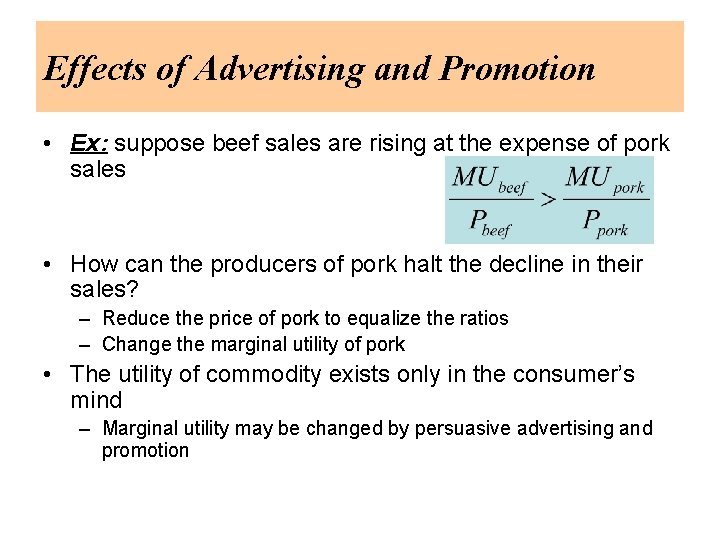Effects of Advertising and Promotion • Ex: suppose beef sales are rising at the expense of pork sales • How can the producers of pork halt the decline in their sales? – Reduce the price of pork to equalize the ratios – Change the marginal utility of pork • The utility of commodity exists only in the consumer’s mind – Marginal utility may be changed by persuasive advertising and promotionMarginal Utility and Demand Curves • A consumer’s demand curve can be derived from marginal utility data • E. g. : • MUM=2; MUX= 200 – 4 QX • Calculating of price from information on marginal utility of commodity X and marginal utility of money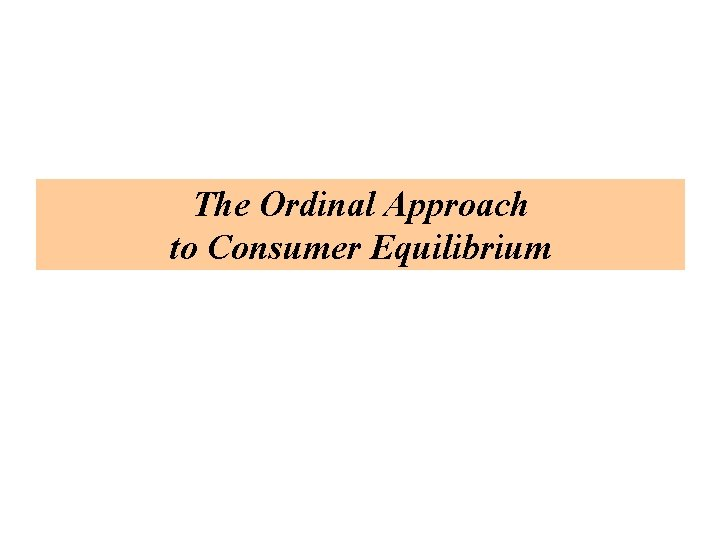The Ordinal Approach to Consumer EquilibriumThe Characteristics of the Consumer’s Preferences • Given three bundles of goods (A, B, and C), if an individual prefers A to B and B to C, he must prefer A to C – If an individual is indifferent between A and B and between B and C, he must be indifferent between A and C • If an individual can rank any pair of bundles chosen at random from all conceivable bundles, he can rank all conceivable bundles • If bundle A contains at least as many units of each commodity as bundle B, and more units of at least one commodity, A must be preferred to B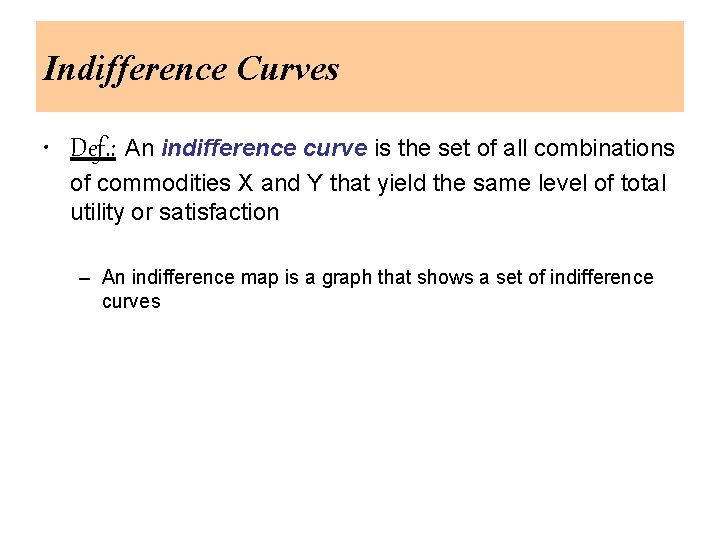Indifference Curves • Def. : An indifference curve is the set of all combinations of commodities X and Y that yield the same level of total utility or satisfaction – An indifference map is a graph that shows a set of indifference curves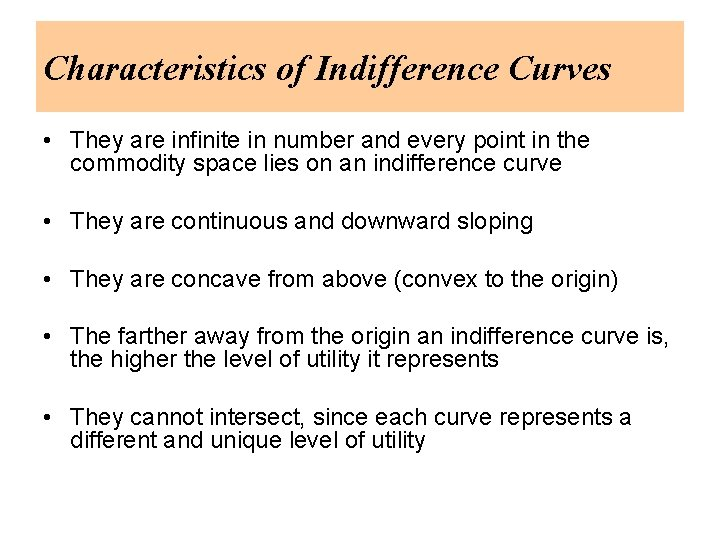Characteristics of Indifference Curves • They are infinite in number and every point in the commodity space lies on an indifference curve • They are continuous and downward sloping • They are concave from above (convex to the origin) • The farther away from the origin an indifference curve is, the higher the level of utility it represents • They cannot intersect, since each curve represents a different and unique level of utility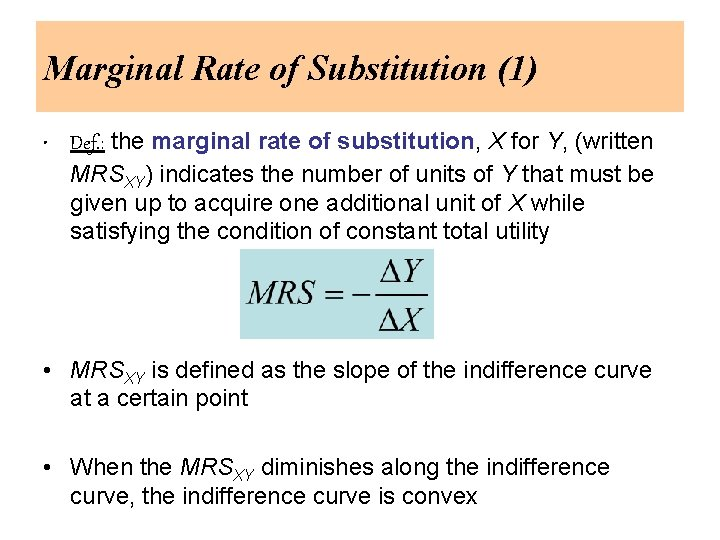Marginal Rate of Substitution (1) • Def. : the marginal rate of substitution, X for Y, (written MRSXY) indicates the number of units of Y that must be given up to acquire one additional unit of X while satisfying the condition of constant total utility • MRSXY is defined as the slope of the indifference curve at a certain point • When the MRSXY diminishes along the indifference curve, the indifference curve is convex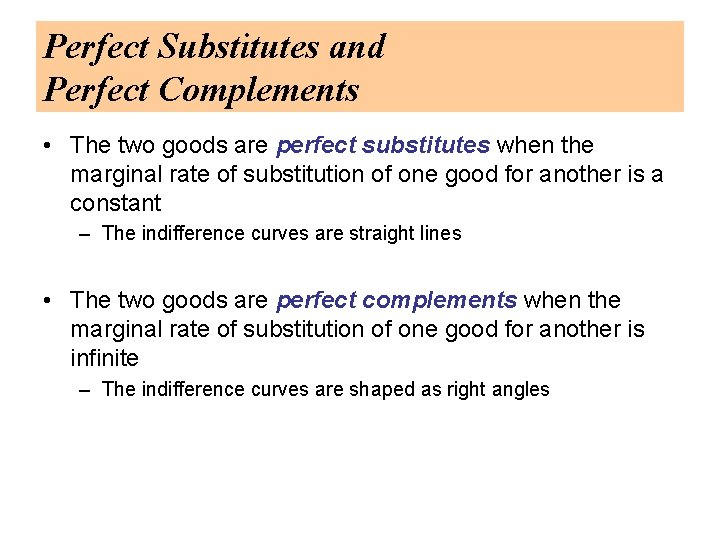Perfect Substitutes and Perfect Complements • The two goods are perfect substitutes when the marginal rate of substitution of one good for another is a constant – The indifference curves are straight lines • The two goods are perfect complements when the marginal rate of substitution of one good for another is infinite – The indifference curves are shaped as right angles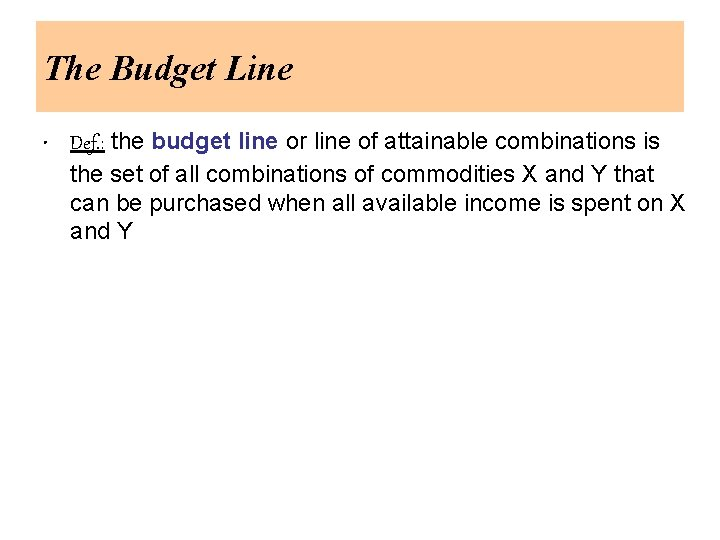The Budget Line • Def. : the budget line or line of attainable combinations is the set of all combinations of commodities X and Y that can be purchased when all available income is spent on X and Y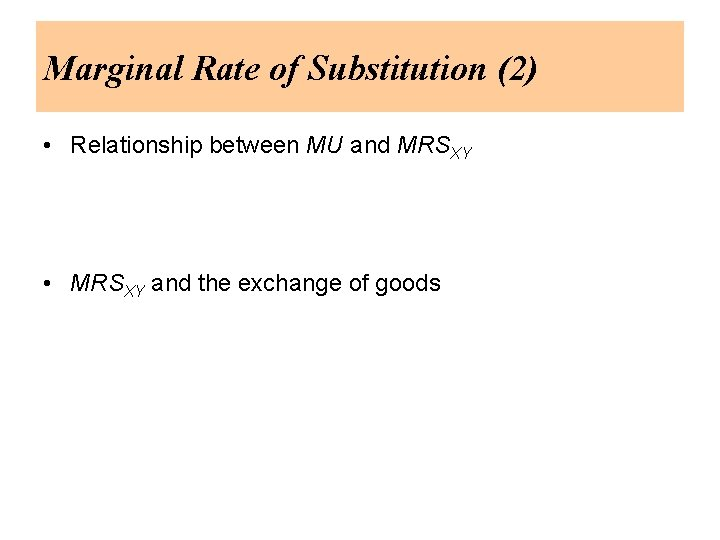Marginal Rate of Substitution (2) • Relationship between MU and MRSXY • MRSXY and the exchange of goods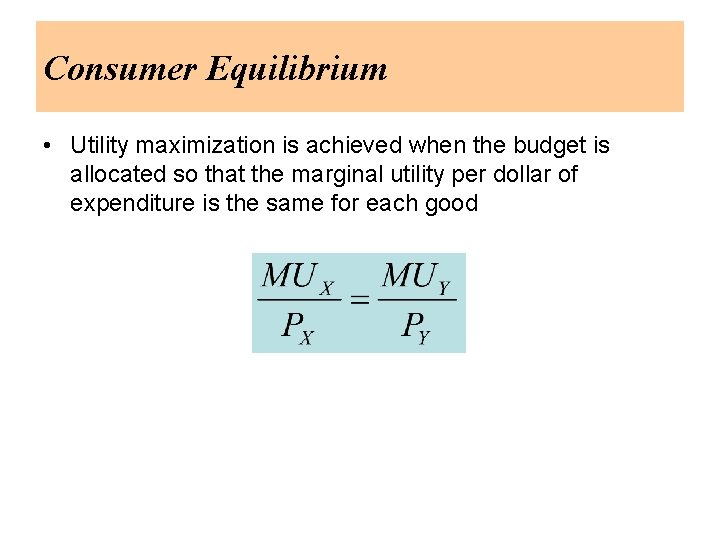Consumer Equilibrium • Utility maximization is achieved when the budget is allocated so that the marginal utility per dollar of expenditure is the same for each good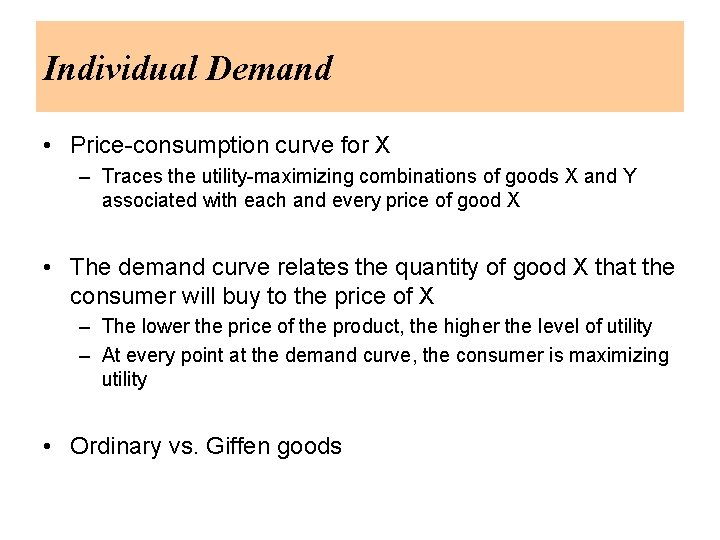Individual Demand • Price-consumption curve for X – Traces the utility-maximizing combinations of goods X and Y associated with each and every price of good X • The demand curve relates the quantity of good X that the consumer will buy to the price of X – The lower the price of the product, the higher the level of utility – At every point at the demand curve, the consumer is maximizing utility • Ordinary vs. Giffen goodsIncome Changes • Income-consumption curve – Traces the utility-maximizing combinations of goods X and Y associated with different levels of income • Engel curves – Relate the quantity of a good consumed to income • Normal vs. inferior goods